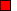WON plate137 | World!OfNumbers[ July 21, 2002 ] 11 ways to arrange the ninedigits Forwarded by Paul Gissing (email) There are eleven ways in which the digits 1, 2, 3, ..., 9 can be arranged as a whole number plus a fraction whose sum is 100. Paul wrote that the question as presented to him stated that there were 11 solutions. One way is96 + (2148/537) = 100 How many others can you find ?[ July 26-27, 2002 ] Jean Claude Rosa (email) wrote that he found a partial solution in the “Théorie des Nombres”  from Edouard Lucas, (18..; he hasn't the exact date of the edition) JCR found the above given example but Edouard Lucas gave only 7 more solutions and not 11. Here they are 100 = 91 + (5742/638) 100 = 91 + (7524/836) 100 = 91 + (5823/647) 100 = 94 + (1578/263)100 = 96 + (2148/537)     100 = 96 + (1428/357) 100 = 96 + (1752/438) One day later JCR came up with the missing 4 thereby completing the initial puzzle. 100 = 3 + (69258/714) 100 = 81 + (5643/297) 100 = 81 + (7524/396) 100 = 82 + (3546/197) JCR likes to ask a few follow-up questions :Exist there also solutions if we replace 100 with another constant ?Resolve the equation P=A+B/C with P prime (or palprime), A and C also prime (of course B must be composite by default) Keeping the spirit of this WONplate A, B and C are written by using once all the digits from 1 to 9.[ August 12, 2002 ] Here are already some solutions from JCR himself. (P is prime and PP is palprime.) 103 = 97 + (2586/431) 639857 = 639851 + (42/7) 958367 = 958361 + (42/7) No solutions for P if digitlength is 7 ! 101 = 97 + (1852/463) 191 = 7 + (85192/463) 13831 = 13597 + (468/2) 94649 = 94531 + (826/7)A000137Prime Curios!Prime PuzzleWikipedia 137Le nombre 137```

```

[TOP OF PAGE]

Patrick De Geest - Belgium- Short Bio - Some Pictures